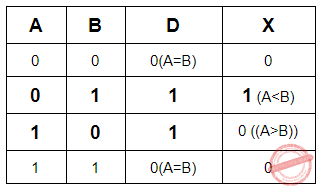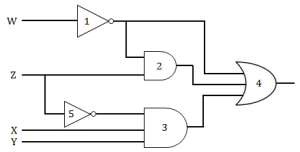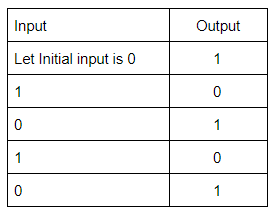## ISRO-2016

 Question 1
Which of the following is true?
 A √3 + √7 = √10 B √3 + √7 ≤ √10 C √3 + √7 < √10 D √3 + √7 > √10
Engineering-Mathematics       Linear-Equation       Video-Explanation
Question 1 Explanation:
√3 + √7=1.7320508075688772935274463415059 + 2.6457513110645905905016157536393
=4.3778021186334678840290620951451
√10=3.1622776601683793319988935444327
So, √3 + √7 > √10 is true.
 Question 2
A given connected graph is a Euler Graph if and only if all vertices of are of
 A same degree B even degree C odd degree D different degree
Engineering-Mathematics       Graph-Theory       Video-Explanation
Question 2 Explanation:
A given connected graph is a Euler Graph if and only if all vertices of are of even degree.
Proof:
→ Let G(V, E) be an Euler graph. Thus G contains an Euler line Z, which is a closed walk.
→ Let this walk start and end at the vertex u ∈ V. Since each visit of Z to an intermediate vertex v of Z contributes two to the degree of v and since Z traverses each edge exactly once, d(v) is even for every such vertex.
→ Each intermediate visit to u contributes two to the degree of u, and also the initial and ﬁnal edges of Z contribute one each to the degree of u. So the degree d(u) of u is also even.
 Question 3
The maximum number of edges in a n-node undirected graph without self loops is
 A n2 B n(n-1)/2 C n-1 D n(n+1)/2
Engineering-Mathematics       Graph-Theory       Video-Explanation
Question 3 Explanation:
The set of vertices has size n, the number of such subsets is given by the binomial coefficient C(n,2)⋅C(n,2)=n(n-1)/2.
 Question 4
The minimum number of NAND gates required to implement the Boolean function A + AB’+ AB’C is equal to
 A 0 B 1 C 4 D 7
Digital-Logic-Design       Boolean-Function       Video-Explanation
Question 4 Explanation:
A + AB’+ AB’C = A(1+B’+B’C) = A
No GATE is required to implement the function A.
 Question 5
The minimum Boolean expression for the following circuit is:A AB + AC + BC B A + BC C A + B D A + B + C
Digital-Logic-Design       Boolean-Expression       Video-Explanation
Question 5 Explanation:
If the switches are in parallel then use “+”, and if they are serial then use “ ”.
A(B+C) + AB + (A+B)C = AB + AC + AB + AC + BC
= (AB+AB) + (AC+AC) + BC
= AB + AC + BC
 Question 6
For a binary half-subtractor having two inputs A and B, the correct set of logical expressions for the outputs D (= A minus B) and X (=borrow) are
 A D = AB + A’B , X = A’B B D = A’B + AB’ , X = AB’ C D = A’B + AB’ , X = A’B D D = AB + A’B , X = AB’
Digital-Logic-Design       Combinational-Circuit       Video-Explanation
Question 6 Explanation:
The function table for the Half Subtractor is as followsA-B= D= A’B + AB’
X= A’B
 Question 7
Consider the following gate networkWhich one of the following gates is redundant?
 A Gate No. 1 B Gate No. 2 C Gate No. 3 D Gate No. 4
Digital-Logic-Design       Logic-Gates       Video-Explanation
Question 7 Explanation:
W’ + W’Z + Z’XY = W’(1+Z) + Z’XY
= W’ + Z’XY
The term W’Z is redundant which is represented by GATE 2.
 Question 8
The dynamic hazard problem occurs in
 A combinational circuit alone B sequential circuit only C Both (a) and (b) D None of the above
Digital-Logic-Design       Hazards       Video-Explanation
Question 8 Explanation:
→ A dynamic hazard is the possibility of an output changing more than once as a result of a single input change.
→ Dynamic hazards often occur in larger logic circuits where there are different routes to the output (from the input).
→ If each route has a different delay, then it quickly becomes clear that there is the potential for changing output values that differ from the required / expected output. e.g.
→ A logic circuit is meant to change output state from 1 to 0, but instead changes from 1 to 0 then 1 and finally rests at the correct value 0. This is a dynamic hazard.
→ As a rule, dynamic hazards are more complex to resolve, but note that if all static hazards have been eliminated from a circuit, then dynamic hazards cannot occur.
 Question 9
The logic circuit given below converts a binary code y1,y2,y3 intoA Excess-3 code B Gray code C BCD code D Hamming Code
Digital-Logic-Design       Number-Systems       Video-Explanation
Question 9 Explanation:
X1= Y1
X2= Y1⊕ Y2
X3= Y2 ⊕ Y3
 Question 10
The circuit given below in the figure below isA An oscillating circuit and its output is a square wave B The one whose output remains stable in ‘1’ state C The one having output remains stable in ‘0’ state D has a single pulse of three times propagation delay
Digital-Logic-Design       Logic-Gates       Video-Explanation
Question 10 Explanation:
The square wave has alternating amplitudes(0 and 1) with duty cycle 1.
An odd number of cascaded NOT gates produce a square wave.Note: Duty cycle= Ratio of durations in which the circuit is ON and OFF in a cycle.
 Question 11
If 12A7C16 = X8, then the value of X is
 A 224174 B 425174 C 6173 D 225174
Digital-Logic-Design       Number-Systems       Video-Explanation
Question 11 Explanation:
Given, (12A7C)16 = (0001 0010 1010 0111 1100)2
MAke blocks of 3 bits each from LSB to MSB.
(Note: In the last block append zeros (as MSBs) if number bits is not three)
(000 010 010 101 001 111 100)
Each of the above blocks represents a digit in base 8 and they can be converted to base 8 as shown below.
= (0 2 2 5 1 7 4)8
 Question 12
The Excess-3 code is also called
 A Cyclic Redundancy Code B Weighted Code C Self-Complementing Code D Algebraic Code
Digital-Logic-Design       Number-Systems       Video-Explanation
Question 12 Explanation:
Excess-3 code is also called Self-Complementing Code. Because 1’s complement of an excess-3 number is equivalent to 9’s complement of the corresponding decimal digit.
→ In excess-3 code, each of the 4-bit numbers represents decimal digit which is 3 less than the actual decimal digit. So the bits have no fixed weight.
Excess-3 code is neither CRC nor Algebraic Code which is used for error detection and/or correction.
 Question 13
The simplified SOP (Sum of Product) form the Boolean expression (P + Q’ + R’)(P + Q’ + R)(P + Q + R’)
 A (P’Q + R) B (P + Q’R’) C (P Q’ + R ) D (PQ + R)
Digital-Logic-Design       Boolean-Expression       Video-Explanation
Question 13 Explanation:Question 14
Which of the following binary number is the same as its 2’s complement?
 A 1010 B 0101 C 1000 D 1001
Digital-Logic-Design       Number-Systems       Video-Explanation
Question 14 Explanation:Hint: Number of bits=4
(Decimal value of maximum 4-bit number +1 )/2= (15+1)/2=8
 Question 15
The functional difference between SR flip-flop and JK flip-flop is that
 A JK Flip-flop is faster than SR flip-flop B JK flip-flop has a feedback path C JK flip-flop accepts both inputs 1 D None of them
Digital-Logic-Design       Sequential-Circuits       Video-Explanation
Question 15 Explanation:
-> JK flip flop accepts input J=K=1. When J=K=1, the state of the flip-flop gets complimented. But it's not a valid input in SR flip-flop.
-> JK flip flop doesn’t have a feedback path.
 Question 16
Consider a non-pipelined processor with a clock rate of 2.5 gigahertz and average cycles per instruction of four. The same processor is upgraded to a pipelined processor with five stages; but due to the internal pipeline delay, the clock speed is reduced to 2 gigahertz. Assume that there are no stalls in the pipeline. The speedup achieved in this pipelined processor is
 A 3.2 B 3 C 2.2 D 2
Computer-Organization       Pipelining       Video-Explanation
Question 16 Explanation:
→ Given that the processor clock rate = 2.5 GHz, the processor takes 2.5 G cycles in one second.
→ Time taken to complete one cycle = (1 / 2.5 G) seconds
→ Since it is given that average number of cycles per instruction = 4, the time taken for completing one instruction=(4/2.5 G) = 1.6 ns
→ In the pipelined case we know in the ideal case CPI = 1, and the clock speed = 2 GHz.
→ Time taken for one instruction in the pipelined case = (1 / 2 G) = 0.5 ns
→ Speedup = 1.6/0.5 = 3.2
 Question 17
What is the output of this C code?A 5 5 5 B 5 5 junk C 5 junk junk D Compile time error
Programming-for-Output-Problems       Pointers       Video-Explanation
Question 17 Explanation:
If we are given p instead of *p then it prints the address of k but we are printing the value of k.
The same rule applies in **m.
Output is 5 5 5
 Question 18
Consider a disk pack with 16 surfaces, 128 tracks per surface and 256 sectors per track. 512 bytes of data are stored in a bit serial manner in a sector. The capacity of the disk pack and the number of bits required to specify a particular sector in the disk are respectively:
 A 256 Mbyte, 19 bits B 256 Mbyte, 21 bits C 512 Mbyte, 20 bits D 64 GB, 28 bits
Computer-Organization       Secondary-Memory       Video-Explanation
Question 18 Explanation:
→ Given that the disk pack has 16 surfaces, 128 tracks per surface, 256 sectors per track and each sector size is 512 bytes.
→ So the disk pack capacity = 16*128*256*512 bytes = 256 MB
→ To specify a sector we need the information about surface number, track number and sector number within a track.
→ Surface number needs 4 bits as there are 16 surfaces(24), track number needs 7 bits as there are 128 tracks(27) within a surface, within a track the sector number needs 8 bits as there are 256 sectors (28).
→ Total number bits needed to specify a particular sector = 4+7+8 = 19 bits.
 Question 19
Let the page fault service time be 10 ms in a computer with average memory access time being 20 ns. If the one-page fault is generated for every 106 memory accesses, what is the effective access time for the memory?
 A 21.4 ns B 29.9 ns C 23.5 ns D 35.1 ns
Operating-Systems       Memory-Management       Video-Explanation
Question 19 Explanation:Question 20
Register renaming is done in pipelined processors
 A as an alternative to register allocation at compile time B for efficient access to function parameters and local variables C to handle certain kinds of hazards D as part of address translation
Computer-Organization       Pipelining       Video-Explanation
Question 20 Explanation:
→ Register renaming is used to eliminate hazards that arise due to WAR (Write After Read) and WAW(Write After Write) dependencies.
There are 20 questions to complete.

Register Now